# Chaos control and Hopf bifurcation analysis of the Genesio system with distributed delays feedback

## Abstract

In this paper, the Genesio system with distributed time delay feedback is investigated. Firstly, the stability of the equilibria of the system is investigated by analyzing the characteristic equation, and then the existence of Hopf bifurcations is verified by choosing the mean time delay as a bifurcation parameter. Subsequent to that, the direction and stability of the bifurcating periodic solutions are determined by using the normal form theory and the center manifold theorem. Finally, some numerical simulations are presented to verify the effectiveness of the theoretical results.

MSC:34K10, 93D15.

## 1 Introduction

Chaos control has attracted considerable attention since the pioneering work of Ott and Grebogi . It is well known that in many practical applications, chaos is undesirable and needs to be controlled. Therefore, the investigation of controlling chaos is of great significance. Many schemes have been presented to implement chaos control, among which using time-delayed controlling forces proves to be a simple and viable method for a continuous dynamical system . It is noteworthy that time-delayed feedback controller can also be used to realize the control of a bifurcation, see  and references therein. It is known that if the steady state is stable or the bifurcating periodic solutions are orbitally asymptotically stable, then the chaotic system will not exhibit chaotic dynamical behaviors. As a consequence, bifurcation control in this sense may also help to control chaos.

In order to better model some complicated practical phenomena, recently, distributed time delay has been introduced into many modeling systems. There are extensive literature works dealing with such systems . As the distributed time delay is incorporated in a system, some interesting dynamical behaviors occur near the equilibrium point. Inspired by these previous works, in this paper, we intend to introduce the distributed time delay as a feedback controller into the chaotic Genesio system with the aim to realize the control of chaos. The rest of this paper is organized as follows. In the next section, we present the mathematical models of the Genesio system with distributed time delay feedback and consider its local stability and Hopf bifurcation. In Section 3, the stability of the bifurcating periodic solutions and the direction of the Hopf bifurcation at the critical values of mean time delay are determined by using the normal form method and the center manifold reduction due to Hassard et al. . In Section 4, a numerical example is provided to verify the theoretical results. Finally, some concluding remarks are given in Section 5.

## 2 Stability analysis and Hopf bifurcation of the Genesio system with distributed delay feedback

The Genesio system, proposed by Genesio and Tesi  and studied extensively in recent years [8, 1419], is described by the following three-dimensional autonomous system

${ x ˙ = y , y ˙ = z , z ˙ = a x + b y + c z + x 2 ,$
(2.1)

where $a,b,c<0$ are parameters. System (2.1) exhibits chaotic dynamical behaviors when $a=−6$, $b=−2.92$, $c=−1.2$, as illustrated in Figure 1.

In order to apply feedback control, we consider system (2.1) with continuous distributed delay feedback described by

${ x ˙ ( t ) = y ( t ) , y ˙ ( t ) = z ( t ) + M ∫ − ∞ 0 ( y ( t ) − y ( t + s ) ) k ( − s ) d s , z ˙ ( t ) = a x ( t ) + b y ( t ) + c z ( t ) + x 2 ( t ) ,$
(2.2)

where $M∈R$, $a,b,c<0$, $∫ 0 + ∞ k(s)ds=1$, $∫ 0 + ∞ sk(s)ds<+∞$.

It is easy to see that systems (2.2) and (2.1) have the same equilibrium points $E 0 (0,0,0)$, $E 1 (−a,0,0)$. Without loss of generality, let $( x ∗ , y ∗ , z ∗ )$ be the equilibrium point of system (2.2), and let $y 1 (t)=x(t)− x ∗$, $y 2 (t)=y(t)− y ∗$, $y 3 (t)=z(t)− z ∗$. Substituting them into system (2.2) yields

${ y ˙ 1 ( t ) = y 2 ( t ) , y ˙ 2 ( t ) = y 3 ( t ) + M y 2 ( t ) − M ∫ − ∞ 0 y 2 ( t + s ) k ( − s ) d s , y ˙ 3 ( t ) = a y 1 ( t ) + b y 2 ( t ) + c y 3 ( t ) + y 1 2 ( t ) + 2 x ∗ y 1 ( t ) .$
(2.3)

Rewrite system (2.3) as follows:

$y ˙ (t)=Ly(t)+ ∫ − ∞ 0 F(s)y(t+s)ds+H(y),$
(2.4)

where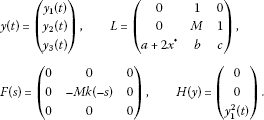The corresponding characteristic equation appears as

$λ(λ−c) ( λ − M + ∫ − ∞ 0 M k ( − s ) e λ s d s ) −bλ− ( a + 2 x ∗ ) =0.$
(2.5)

In this paper, we consider the weak kernel case , i.e., $k(s)=α e − α s$, where $α>0$. The analysis for the general gamma kernel case is similar. We define the initial condition of system (2.3) as follows:

$( y 1 ( s ) y 2 ( s ) y 3 ( s ) )=( ϕ 1 ( s ) ϕ 2 ( s ) ϕ 3 ( s ) ),−∞

The characteristic equation (2.5) under the weak kernel case then takes the form

$λ 4 + n 1 (α) λ 3 + n 2 (α) λ 2 + n 3 (α)λ+ n 4 (α)=0,$
(2.6)

where

${ n 1 ( α ) = α − M − c , n 2 ( α ) = M c − c α − b , n 3 ( α ) = − b α − a − 2 x ∗ , n 4 ( α ) = − ( a + 2 x ∗ ) α .$

It follows from the well-known Routh-Hurwitz criterion that all the roots of Eq. (2.6) have negative real parts if the following conditions are satisfied:

${ D 1 ( α ) ≡ n 1 ( α ) = α − M − c > 0 , D 2 ( α ) ≡ n 1 ( α ) n 2 ( α ) − n 3 ( α ) = ( α − M − c ) ( M c − c α − b ) + b α + a + 2 x ∗ > 0 , D 3 ( α ) ≡ n 3 ( α ) D 2 ( α ) − n 1 2 ( α ) n 4 ( α ) = ( − b α − a − 2 x ∗ ) [ ( α − M − c ) ( M c − c α − b ) D 3 ( α ) ≡ + b α + a + 2 x ∗ ] + α ( a + 2 x ∗ ) ( α − M − c ) 2 > 0 , D 4 ( α ) ≡ n 4 ( α ) D 3 ( α ) > 0 .$

It is easy to see that at $E 1 (−a,0,0)$, we always have $n 4 (a)=aα<0$, thus the equilibrium point $E 1 (−a,0,0)$ is unstable. In what follows, we only analyze the equilibrium point $E 0 (0,0,0)$. Straightforwardly, we have the following result.

Theorem 1 The equilibrium point $E 0 (0,0,0)$ of system (2.2), where $k(s)$ represents the weak kernel, is locally asymptotically stable if the following conditions hold:

${ α − M − c > 0 , ( α − M − c ) ( M c − c α − b ) + b α + a > 0 , ( − b α − a ) [ ( α − M − c ) ( M c − c α − b ) + b α + a ] + a α ( α − M − c ) 2 > 0 .$
(2.7)

Let $λ i$ ($i=1,2,3,4$) be the roots of Eq. (2.6), then we have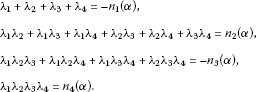If there exists an $α 0 ∈ R +$ such that $D 3 ( α 0 )=0$ and $d D 3 (α)/dα | α = α 0 ≠0$, then by the Routh-Hurwitz criterion, there exists a pair of purely imaginary roots, say $λ 1 = λ 2 ¯ =i ω 0$ ($ω 0 ≠0$), and the other two roots $λ 3$, $λ 4$ satisfy: if $λ 3$, $λ 4$ are real, then $λ 3 <0$, $λ 4 <0$; if $λ 3$, $λ 4$ are complex conjugate, then $Re λ 3 =Re λ 4 =− n 1 (α)/2$. It is easy to calculate that

$d ( Re λ 1 ) d α | α 0 =− n 1 ( α ) 2 [ n 1 3 ( α ) n 3 ( α ) + ( n 1 ( α ) n 2 ( α ) − 2 n 3 ( α ) ) 2 ] ⋅ d D 3 ( α ) d α | α 0 ,$

thus the Hopf bifurcation occurs at $E 0$ as α passes through $α 0$.

## 3 Direction and stability of bifurcating periodic solutions

In this section, we investigate the direction, stability and period of bifurcating periodic solutions from the steady state by applying the normal form theory and the center manifold theorem developed by Hassard et al. in . Let $μ=α− α 0$, then system (2.3) undergoes the Hopf bifurcation at $E 0 (0,0,0)$ near $μ=0$. Assume that $±i ω 0$ is the corresponding purely imaginary roots of Eq. (2.6) at steady state $E 0 (0,0,0)$ for $μ=0$. We transform system (2.4) into an FDE in $C((−∞,0], R 3 )$ as

$y ˙ t =A(μ) y t +R(μ) y t ,$
(3.1)

where $y t (θ)=y(t+θ)$, $θ∈(−∞,0)$, $y= ( y 1 , y 2 , y 3 ) T$, and operators A and R are defined as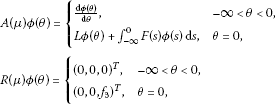where

$f 3 = ϕ 1 2 (0).$

For $ψ∈C([0,+∞), ( R 3 ) ∗ )$, $ψ(s)= ( ψ 1 ( s ) , ψ 2 ( s ) , ψ 3 ( s ) ) T ∈C[0,+∞)$, the adjoint operator of A denoted by $A ∗$ is defined as

$A ∗ (μ)ψ(s)={ − d ψ ( s ) d s , 0 < s < + ∞ , L T ψ ( 0 ) + ∫ − ∞ 0 F T ( t ) ψ ( − t ) d t , s = 0 .$
(3.2)

For $ϕ∈C(−∞,0]$ and $ψ∈C[0,+∞)$, a bilinear inner product is defined as

$〈ψ,ϕ〉= ψ ¯ T (0)ϕ(0)− ∫ θ = − ∞ 0 ∫ ξ = 0 θ ψ ¯ T (ξ−θ)F(θ)ϕ(ξ)dξdθ.$

In what follows, we need to calculate the eigenvector q of A associated with the eigenvalue $i ω 0$ and the eigenvector $q ∗$ of $A ∗$ associated with the eigenvalue $−i ω 0$. Assume that $q(θ)= ( 1 , β , γ ) T e i ω 0 θ$ is the eigenvector of $A(0)$ corresponding to $i ω 0$, then $A(0)q(0)=i ω 0 q(0)$, namely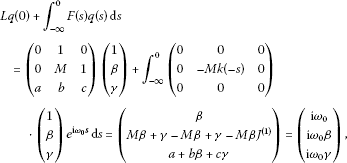where

$J ( 1 ) = ∫ − ∞ 0 k(−s) e i ω 0 s ds= α α + i ω 0 .$

It is easy to calculate from the above equality that

${ β = i ω 0 , γ = α + i ω 0 b i ω 0 − c = − ω 0 2 − i ω 0 M + i ω 0 M J ( 1 ) .$

Assume that $q ∗ (ζ)=N ( 1 , β ∗ , γ ∗ ) T e i ω 0 ζ$, $0≤ζ<+∞$, then $A ∗ (0) q ∗ (0)=−i ω 0 q ∗ (0)$, that is,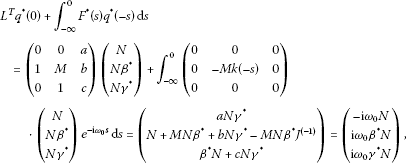where

$J ( − 1 ) = ∫ − ∞ 0 k(−s) e − i ω 0 s ds= α α − i ω 0 .$

Hence we have

${ β ∗ = ω 0 2 + i ω 0 c a = a − i ω 0 a ( i ω 0 − M + M J ( − 1 ) ) , γ ∗ = − i ω 0 a .$

We choose $N= 1 1 + β ∗ β ¯ + γ ∗ γ ¯ + M β ∗ β ¯ ∫ θ = − ∞ 0 θ e − i ω 0 θ k ( − θ ) d θ$, then $〈 q ∗ ,q〉=1$, $〈 q ∗ , q ¯ 〉=0$ hold. In what follows, we follow the same notations as in . We first construct the coordinates of the center manifold $Ω 0$ at $μ=0$. Let

$z(t)= 〈 q ∗ , u t 〉 ,w(t,θ)= u t −2Re { z ( t ) q ( θ ) } .$

On the center manifold $Ω 0$, we have

$w(t,θ)=w(z, z ¯ ,θ),$

where

$w(z, z ¯ ,θ)= w 20 (θ) z 2 2 + w 11 (θ)z z ¯ + w 02 (θ) z ¯ 2 2 +⋯,$
(3.3)

and z, $z ¯$ are the local coordinates of the center manifold $Ω 0$ in the directions of $q ∗$ and $q ¯ ∗$ respectively.

Note that w is real if $u t$ is real. We only consider real solutions. For the solution $u t ∈ Ω 0$ of (3.1), since $μ=0$, we have

$z ˙ ( t ) = i ω 0 z ( t ) + 〈 q ∗ , R ( w + 2 Re { z ( t ) q ( θ ) } ) 〉 = i ω 0 z ( t ) + q ∗ ¯ T ( 0 ) R ( w ( z , z ¯ , 0 ) + 2 Re { z ( t ) q ( 0 ) } ) .$

Rewrite the above equation as

$z ˙ (t)=i ω 0 z(t)+g(z, z ¯ ),$

where

$g(z, z ¯ )= q ∗ ¯ T (0)R ( w ( z , z ¯ , 0 ) + 2 Re { z ( t ) q ( 0 ) } ) .$
(3.4)

Expand the function $g(z, z ¯ )$ on the center manifold $Ω 0$ as

$g(z, z ¯ )= g 20 z 2 2 + g 11 z z ¯ + g 02 z ¯ 2 2 + g 21 z 2 z ¯ 2 +⋯.$
(3.5)

By (3.1) and (3.3), we have

$w ˙ = u ˙ t − z ˙ q− z ¯ ˙ q ¯ =Aw−2Re { g ( z , z ¯ ) q ( θ ) } +R ( w + 2 Re { z ( t ) q ( θ ) } ) .$

Rewrite this as

$w ˙ =Aw+H(z, z ¯ ,θ),$

where

$H(z, z ¯ ,θ)=−2Re { g ( z , z ¯ ) q ( θ ) } +R ( w + 2 Re { z ( t ) q ( θ ) } ) .$
(3.6)

Expand the function $H(z, z ¯ ,θ)$ on the center manifold $Ω 0$ as

$H(z, z ¯ ,θ)= H 20 (θ) z 2 2 + H 11 (θ)z z ¯ + H 02 (θ) z ¯ 2 2 +⋯.$

While

$w+zq(θ)+ z ¯ q ¯ (θ)=( w ( 1 ) ( θ ) + z e i ω 0 θ + z ¯ e − i ω 0 θ w ( 2 ) ( θ ) + z β e i ω 0 θ + z ¯ β ¯ e − i ω 0 θ w ( 3 ) ( θ ) + z γ e i ω 0 θ + z ¯ γ ¯ e − i ω 0 θ ).$

Thus

$R ( w + 2 Re { z ( t ) q ( θ ) } ) ={ ( 0 0 0 ) , − ∞ < θ < 0 , ( 0 0 f 0 ( 3 ) ) , θ = 0 ,$

where

$f 0 ( 3 ) = [ w ( 1 ) ( 0 ) + z + z ¯ ] 2 .$

It follows from (3.4) that

$g(z, z ¯ )= N ¯ ( 1 , β ∗ ¯ , γ ∗ ¯ ) ( 0 0 f 0 ( 3 ) )= N ¯ γ ∗ ¯ f 0 ( 3 ) (z, z ¯ ).$

Hence

$H(z, z ¯ ,θ)=−2Re { N ¯ γ ∗ ¯ f 0 ( 3 ) ( z , z ¯ ) q ( θ ) } +{ ( 0 0 0 ) , − ∞ < θ < 0 , ( 0 0 f 0 ( 3 ) ) , θ = 0 .$

From (3.4), we obtain

$H 20 (θ)=−2Re { N ¯ γ ∗ ¯ f 0 , z 2 ( 3 ) q ( θ ) } +{ ( 0 0 0 ) , − ∞ < θ < 0 , ( 0 0 f 0 , z 2 ( 3 ) ) , θ = 0 .$

Notice that $f 0 , z 2 ( 3 ) =2$, we have

$H 20 (θ)=−4Re { N ¯ γ ∗ ¯ q ( θ ) } +{ ( 0 0 0 ) , − ∞ < θ < 0 , ( 0 0 2 ) , θ = 0 .$
(3.7)

Similarly, we have

$H 11 (θ)=4Re { N ¯ γ ∗ ¯ q ( θ ) } +{ ( 0 0 0 ) , − ∞ < θ < 0 , ( 0 0 2 ) , θ = 0 .$

On the other hand, on the center manifold $Ω 0$ near the origin, we have

$w ˙ (z, z ¯ )= w z z ˙ + w z ¯ z ¯ ˙ .$

Expanding the above equation and comparing the corresponding coefficients, we get

$( A − 2 i ω 0 I ) w 20 ( θ ) = − H 20 ( θ ) , A w 11 ( θ ) = − H 11 ( θ ) .$
(3.8)

Define

$w 20 (θ)=( w 20 ( 1 ) ( θ ) w 20 ( 2 ) ( θ ) w 20 ( 3 ) ( θ ) ),−∞<θ<0.$

Substituting (3.2) and (3.7) into (3.8), when $−∞<θ<0$, we have

$( 2 i ω 0 − d d θ 0 0 0 2 i ω 0 − d d θ 0 0 0 2 i ω 0 − d d θ )( w 20 ( 1 ) ( θ ) w 20 ( 2 ) ( θ ) w 20 ( 3 ) ( θ ) )=( − 4 Re { N ¯ γ ∗ ¯ e i ω 0 θ } − 4 Re { N ¯ γ ∗ ¯ β e i ω 0 θ } − 4 Re { N ¯ γ ∗ ¯ γ e i ω 0 θ } ).$
(3.9)

When $θ=0$, we obtain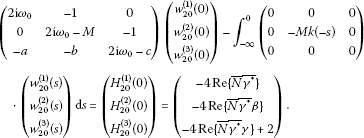(3.10)

In order to guarantee the continuity of solutions, we further assume that $( w 20 ( 1 ) ( θ ) w 20 ( 2 ) ( θ ) w 20 ( 3 ) ( θ ) )$ is continuous at $θ=0$.

It follows from (3.9) that

${ d w 20 ( 1 ) ( θ ) d θ = 2 i ω 0 w 20 ( 1 ) ( θ ) + 4 Re { N ¯ γ ∗ ¯ e i ω 0 θ } , d w 20 ( 2 ) ( θ ) d θ = 2 i ω 0 w 20 ( 2 ) ( θ ) + 4 Re { N ¯ γ ∗ ¯ β e i ω 0 θ } , d w 20 ( 3 ) ( θ ) d θ = 2 i ω 0 w 20 ( 3 ) ( θ ) + 4 Re { N ¯ γ ∗ ¯ γ } − 2 .$

The solutions of the above equations take the form

$( w 20 ( 1 ) ( θ ) w 20 ( 2 ) ( θ ) w 20 ( 3 ) ( θ ) )=( l 2 m 2 n 2 ) e 2 i ω 0 θ +( l 1 m 1 n 1 ) e i ω 0 θ +( l 0 m 0 n 0 ) e − i ω 0 θ ,$
(3.11)

where

${ l 0 = 8 N γ ∗ e − i ω 0 θ 3 i ω 0 , m 0 = β l 0 , n 0 = γ l 0 , { l 1 = 8 N ¯ γ ∗ ¯ e i ω 0 θ i ω 0 , m 1 = β l 1 , n 1 = γ l 1 , { l 2 = w 20 ( 1 ) ( 0 ) − l 0 − l 1 , m 2 = w 20 ( 2 ) ( 0 ) − m 0 − m 1 , n 2 = w 20 ( 3 ) ( 0 ) − n 0 − n 1 .$

Substituting (3.11) into (3.10) yields

$( 2 i ω 0 − 1 0 0 2 i ω 0 − M + M J ( 2 ) − 1 − a − b 2 i ω 0 − c )( w 20 ( 1 ) ( 0 ) w 20 ( 2 ) ( 0 ) w 20 ( 3 ) ( 0 ) )=( G 20 ( 1 ) G 20 ( 2 ) G 20 ( 13 ) ),$

where

${ G 20 ( 1 ) = H 20 ( 1 ) ( 0 ) , G 20 ( 2 ) = H 20 ( 2 ) ( 0 ) + M [ ( m 1 + m 0 ) J ( 2 ) − m 1 J ( 1 ) − m 0 J ( − 1 ) ] , G 20 ( 3 ) = H 20 ( 3 ) ( 0 ) ,$

and

$J ( 2 ) = ∫ − ∞ 0 k(−s) e 2 i ω 0 s ds= α α + 2 i ω 0 .$

Let

$B − 1 =( 2 i ω 0 − 1 0 0 2 i ω 0 − M + M J ( 2 ) − 1 − a − b 2 i ω 0 − c ).$

Then

$B= 1 Λ ( B 11 B 12 B 13 B 21 B 22 B 23 B 31 B 32 B 33 ),$

where $Λ=det( B − 1 )=−8i ω 0 3 +4(c−M+M J ( 2 ) ) ω 0 2 +2i(Mc−M J ( 2 ) −b) ω 0 −a$, and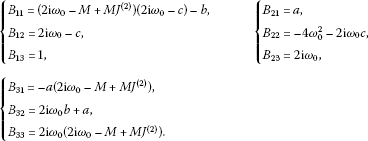Therefore, the following can be determined:

$( w 20 ( 1 ) ( 0 ) w 20 ( 2 ) ( 0 ) w 20 ( 3 ) ( 0 ) )= 1 Λ ( B 11 G 20 ( 1 ) + B 12 G 20 ( 2 ) + B 13 G 20 ( 3 ) B 21 G 20 ( 1 ) + B 22 G 20 ( 2 ) + B 23 G 20 ( 3 ) B 31 G 20 ( 1 ) + B 32 G 20 ( 2 ) + B 33 G 20 ( 3 ) ).$

Following the similar analysis presented above, we have

$( w 11 ( 1 ) ( θ ) w 11 ( 2 ) ( θ ) w 11 ( 3 ) ( θ ) )=( p 2 q 2 r 2 )+( p 1 q 1 r 1 ) e i ω 0 θ +( p 0 q 0 r 0 ) e − i ω 0 θ ,$

where

${ p 0 = 2 N γ ∗ i ω 0 , q 0 = β p 0 , r 0 = γ p 0 , { p 1 = − 2 N ¯ γ ∗ ¯ i ω 0 , q 1 = β p 1 , r 1 = γ p 1 , { p 2 = w 11 ( 1 ) ( 0 ) − p 0 − p 1 , q 2 = w 11 ( 2 ) ( 0 ) − q 0 − q 1 , r 2 = w 11 ( 3 ) ( 0 ) − r 0 − r 1 .$

The following can be calculated:

$( w 11 ( 1 ) ( 0 ) w 11 ( 2 ) ( 0 ) w 11 ( 3 ) ( 0 ) )= 1 − a ( C 11 G 11 ( 1 ) + C 12 G 11 ( 2 ) + C 13 G 11 ( 3 ) C 21 G 11 ( 1 ) + C 22 G 11 ( 2 ) + C 23 G 11 ( 3 ) C 31 G 11 ( 1 ) + C 32 G 11 ( 2 ) + C 33 G 11 ( 3 ) ),$

where

${ G 11 ( 1 ) = H 11 ( 1 ) ( 0 ) , G 11 ( 2 ) = H 11 ( 2 ) ( 0 ) + M [ q 0 + q 1 − q 0 J ( − 1 ) − q 1 J ( 1 ) ] , G 11 ( 3 ) = H 11 ( 3 ) ( 0 ) ,$

and

${ C 11 = M c − M J ( 2 ) c , C 12 = − c , C 13 = 1 , { C 21 = a , C 22 = 0 , C 23 = 0 , { C 31 = − M a + M J ( 2 ) a , C 32 = a , C 33 = 0 .$

Next, we consider $R(w(z, z ¯ ,0)+2Re{z(t)q(0)})$, noticing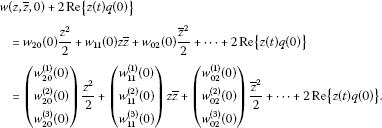While

$f 0 ( 3 ) = [ w 20 ( 1 ) ( 0 ) z 2 2 + w 11 ( 1 ) ( 0 ) z z ¯ + w 02 ( 1 ) ( 0 ) z ¯ 2 2 + ⋯ + z + z ¯ ] 2 .$

Hence

$g ( z , z ¯ ) = q ∗ ¯ T ( 0 ) R ( w ( z , z ¯ , 0 ) + 2 Re { z ( t ) q ( 0 ) } ) = N ¯ ( 1 , β ∗ ¯ , γ ∗ ¯ ) ( 0 0 f 0 ( 3 ) ) = N ¯ γ ∗ ¯ f 0 ( 3 ) .$

Comparing the coefficients with (3.5), we obtain

$g 20 = 2 N ¯ γ ∗ ¯ , g 11 = 2 N ¯ γ ∗ ¯ , g 02 = 2 N ¯ γ ∗ ¯ , g 21 = 2 N ¯ γ ∗ ¯ ( w 20 ( 1 ) ( 0 ) + 2 w 11 ( 1 ) ( 0 ) ) .$

Therefore, the following values can be calculated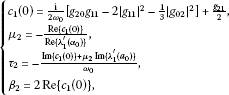(3.12)

which determine the quantities of bifurcating periodic solutions on the center manifold $Ω 0$ at the critical value $α 0$, i.e., $μ 2$ determines the directions of the Hopf bifurcation: if $μ 2 >0$ ($μ 2 <0$), then the Hopf bifurcation is supercritical (subcritical) and the bifurcating periodic solutions exist for $α> α 0$; $τ 2$ determines the period of the bifurcating periodic solutions: the period increases (decreases) if $τ 2 >0$ ($τ 2 <0$); $β 2$ determines the stability of the bifurcating periodic solutions: the bifurcating periodic solutions are stable (unstable) if $β 2 <0$ ($β 2 >0$).

## 4 Numerical simulations

In this section, we shall perform some numerical simulations to verify the analytical results presented in the previous sections. Let us take $a=−6$, $b=−2.92$, $c=−1.2$, $M=−1$ in system (2.2) and consider the weak kernel case, i.e.,

${ x ˙ ( t ) = y ( t ) , y ˙ ( t ) = z ( t ) − ∫ − ∞ 0 ( y ( t ) − y ( t + s ) ) k ( − s ) d s , z ˙ ( t ) = − 6 x ( t ) − 2.92 y ( t ) − 1.2 z ( t ) + x 2 ( t ) ,$
(4.1)

with $k(s)=α e − α s$, $α>0$. The initial conditions are given as $x(0)=0.3$, $y(0)=0.1$, $z(0)=0.3$; $y(t≤0)=0.1$. We can easily determine by these parameters that $α 0 ≐1.40017$. For a very small α, when $α=0.00001$, we see in Figure 2 that the attractor of system (4.1) still exists. But when $α=1.3<1.40017$, as all the conditions in (2.7) are satisfied, the equilibrium point $E 0 (0,0,0)$ in system (4.1) is asymptotically stable, which is illustrated in Figure 3. When $α= α 0 ≐1.40017$, system (2.2) undergoes the Hopf bifurcation at $E 0 (0,0,0)$, as illustrated in Figure 4. Moreover, from the formulae (3.12) presented in Section 3, it follows that $μ 2 >0$, $β 2 <0$, $τ 2 >0$, the Hopf bifurcation is supercritical and the direction of the bifurcation is $α> α 0$. However, as α increases, when $α=1.5>1.40017$, the third condition in (2.7) does not hold, hence the equilibrium point $E 0 (0,0,0)$ in system (4.1) is unstable, as illustrated in Figure 5.

As compared with the former method, a chaotic model with distributed delay feedback is more general than that with discrete delay feedback , because the distributed delay becomes a discrete delay when the delay kernel is a delta function at a certain time. The distributed delay has found widespread applications in many fields such as neural network [8, 10], complicated real models , the modeling of aggregative processes involving the flow of entities with random transit times through a given process , and so on. Therefore, it is of considerable significance to propose distributed delays as control input to control the chaotic system.

From the numerical simulations, we see, as the distributed delay feedback is incorporated in the chaotic Genesio system, a rich spectrum of dynamical behaviors can occur by adjusting the mean time delay values. Chaotic behaviors vanish and the orbitally asymptotically stable Hopf bifurcation occurs as the mean time delay reaches a certain value. Also, we can determine the critical mean time delay value that the Hopf bifurcation occurs at, which is of great help when choosing appropriate parameter values to realize Hopf bifurcation control.

## 5 Concluding remarks

In this paper, the Genesio system with distributed time delay feedback has been studied. It has been demonstrated that the Hopf bifurcation occurs near the steady state as the average time delay crosses the critical value. The explicit formulae for determining the direction, stability and period of bifurcating periodic solutions have been presented by using the normal form theory and the center manifold theorem. A numerical example is provided to verify the theoretical results.

## References

1. 1.

Ott E, Grebogi C, Yorke JA: Controlling chaos. Phys. Rev. Lett. 1990, 64: 1196–1199. 10.1103/PhysRevLett.64.1196

2. 2.

Pyragas K: Continuous control of chaos by selfcontrolling feedback. Phys. Lett. A 1992, 170: 421–428. 10.1016/0375-9601(92)90745-8

3. 3.

Song Y, Wei J: Bifurcation analysis for Chen’s system with delayed feedback and its application to control of chaos. Chaos Solitons Fractals 2004, 22: 75–91. 10.1016/j.chaos.2003.12.075

4. 4.

Guo S, Feng G, Liao X, Liu Q: Hopf bifurcation control in a congestion control model via dynamic delayed feedback. Chaos 2008., 18: Article ID 043104

5. 5.

Gakkhar S, Singh A: Complex dynamics in a prey predator system with multiple delays. Commun. Nonlinear Sci. Numer. Simul. 2012, 17: 914–922. 10.1016/j.cnsns.2011.05.047

6. 6.

Atay FM: Delayed feedback control near Hopf bifurcation. Discrete Contin. Dyn. Syst., Ser. S 2008, 1: 197–205.

7. 7.

Liao X, Chen G: Hopf bifurcation and chaos analysis of Chen’s system with distributed delays. Chaos Solitons Fractals 2005, 25: 197–220. 10.1016/j.chaos.2004.11.007

8. 8.

Zhou L, Chen F: Hopf bifurcation and Si’lnikov chaos of Genesio system. Chaos Solitons Fractals 2009, 40: 1413–1422. 10.1016/j.chaos.2007.09.033

9. 9.

Balachandran K, Zhou Y, Kokila J: Relative controllability of fractional dynamical systems with delays in control. Commun. Nonlinear Sci. Numer. Simul. 2012, 17: 3508–3520. 10.1016/j.cnsns.2011.12.018

10. 10.

Zhao H, Wang L: Hopf bifurcation in Cohen-Grossberg neural network with distributed delays. Nonlinear Anal., Real World Appl. 2007, 8: 73–89. 10.1016/j.nonrwa.2005.06.002

11. 11.

Adimy M, Crauste F, Ruan S: Stability and Hopf bifurcation in a mathematical model of pluripotent stem cell dynamics. Nonlinear Anal., Real World Appl. 2005, 6: 651–670. 10.1016/j.nonrwa.2004.12.010

12. 12.

Hassard B, Kazarinoff N, Wan Y: Theory and Applications of Hopf Bifurcation. Cambridge University Press, Cambridge; 1981.

13. 13.

Genesio R, Tesi A: Harmonic balance methods for analysis of chaotic dynamics in nonlinear systems. Automatica 1992, 28: 531–548. 10.1016/0005-1098(92)90177-H

14. 14.

Park JH: Further results on functional projective synchronization of Genesio-Tesi chaotic system. Mod. Phys. Lett. B 2009, 24(15):1889–1895.

15. 15.

Park JH: Exponential synchronization of the Genesio-Tesi chaotic system via a novel feedback control. Phys. Scr. 2007, 76(6):617–622. 10.1088/0031-8949/76/6/004

16. 16.

Sun Y: Chaos synchronization of uncertain Genesio-Tesi chaotic systems with deadzone nonlinearity. Phys. Lett. A 2009, 373: 3273–3276. 10.1016/j.physleta.2009.07.025

17. 17.

Park JH, Lee SM, Kwon OM: Adaptive synchronization of Genesio-Tesi chaotic system via a novel feedback control. Phys. Lett. A 2007, 371(4):263–270. 10.1016/j.physleta.2007.06.020

18. 18.

Park JH, Kwon OM, Lee SM: LMI optimization approach to stabilization of Genesio-Tesi chaotic system via dynamic controller. Appl. Math. Comput. 2008, 196(1):200–206. 10.1016/j.amc.2007.05.045

19. 19.

Wang G: Stabilization and synchronization of Genesio-Tesi system via single variable feedback controller. Phys. Lett. A 2010, 374: 2831–2834. 10.1016/j.physleta.2010.05.007

20. 20.

Manetsch TJ: Time-varying distributed delays and their use in aggregative models of large systems. IEEE Trans. Syst. Man Cybern. 1976, 6(8):547–553.

## Acknowledgements

The authors would like to thank the anonymous referees for their valuable comments. This work was supported by the National Natural Science Foundation of China (No. 11171084, No. 61273077) and the Natural Science Foundation of Zhejiang Province (No. LY12A01001).

## Author information

Authors

### Corresponding author

Correspondence to Junbiao Guan.

### Competing interests

The authors declare that they have no competing interests.

### Authors’ contributions

JG drafted the manuscript. FC and GW checked the writing and revised the manuscript. All authors read and approved the final manuscript.

## Authors’ original submitted files for images

Below are the links to the authors’ original submitted files for images.

## Rights and permissions

Reprints and Permissions

Guan, J., Chen, F. & Wang, G. Chaos control and Hopf bifurcation analysis of the Genesio system with distributed delays feedback. Adv Differ Equ 2012, 166 (2012). https://doi.org/10.1186/1687-1847-2012-166